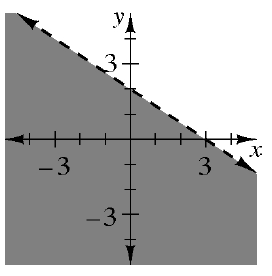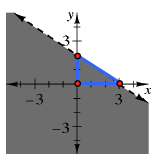### Home > CCAA8 > Chapter 10 Unit 11 > Lesson CCA: 10.2.1 > Problem10-31

10-31.

Write the inequality represented by the graph at right.Use a slope triangle to find the slope of the line.
$\text{Slope}=-\frac{2}{3}$

Find the $y$-intercept on the graph. $\left(0,2\right)$Now substitute the slope and the $y$-intercept into the Slope-Intercept form of a line. $y=mx+b$
$y=-\frac{2}{3}+2$

Replace the '$=$' with a '$<$' sign, as the graph has the portion below the line shaded.

$y<-\frac{2}{3}x+2$